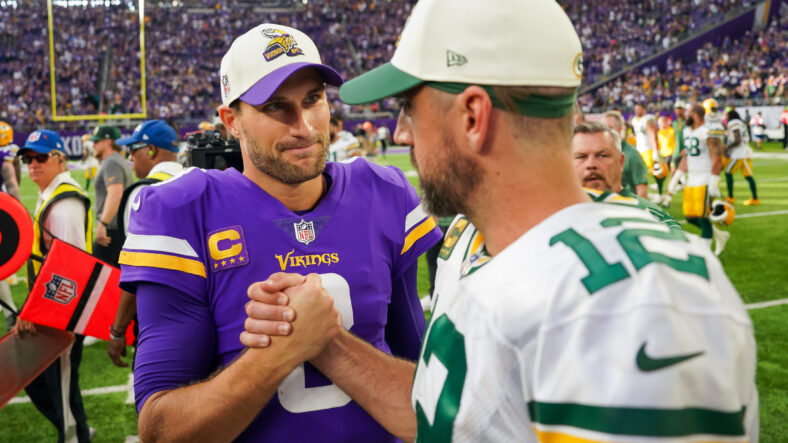# NFL’s Top QBs after Week 1: PFF, DVOA, EPA+CPOE FormulaNFL’s Top QBs after Week 1: PFF, DVOA, EPA+CPOE Formula

The following scores are “good” through September 14th, 2022.

This metric merges quarterback grades and scores from Pro Football Focus, FootballOutsiders’ DVOA, EPA+CPOE, and the traditional Passer Rating. The objective is to rank quarterbacks week-by-week with a progressive rolling tally and set of rankings.

The following scores and rankings are weighted and scaled to 100. PFF accounts for 30% of the score, DVOA with 30%, EPA+CPOE at 30%, and Passer Rating 10%. Skill position players and players with menial pass attempts were excluded.

These are the NFL’s top quarterbacks after Week 1.

32. Dak Prescott (DAL)3.9
PFF = 37.4, EPA+CPOE = -0.093, DVOA = -52.3, PR = 47.2

31. Trey Lance (SF)13.5
PFF = 53.7, EPA+CPOE = -0.081, DVOA = -44.9, PR = 50.3

30. Justin Fields (CHI)21.2
PFF = 44.2, EPA+CPOE = .011, DVOA = -43.7, PR = 85.7

29. Derek Carr (LV)21.7
PFF = 41.5, EPA+CPOE = .014, DVOA = -29.9, PR = 69.1Sep 12, 2021; Cincinnati, Ohio, USA; Cincinnati Bengals quarterback Joe Burrow. Mandatory Credit: Joseph Maiorana-USA TODAY Sports.

28. Joe Burrow (CIN)22.3
PFF = 53.7, EPA+CPOE = .02, DVOA = -48.1, PR = 61.7

27. Baker Mayfield (CAR)24.5
PFF = 47.0, EPA+CPOE = .016, DVOA = -35.4, PR = 84.6

26. Matthew Stafford (LAR)25.3
PFF = 64.7, EPA+CPOE = .023, DVOA = -56.4, PR = 63.1

25. Aaron Rodgers (GB)28.1
PFF = 60.1, EPA+CPOE = .013, DVOA = -34.2, PR = 67.6

24. Jacoby Brissett (CLE)31.3
PFF = 46.9, EPA+CPOE = .027, DVOA = -4.1, PR = 74.0

23. Mitchell Trubisky (PIT)32.0
PFF = 62.0, EPA+CPOE = -.013, DVOA = -15.9, PR = 78.2

22. Jared Goff (DET)32.8
PFF = 49.5, EPA+CPOE = .014, DVOA = -0.3, PR = 80.3

21. Joe Flacco (NYJ)36.9
PFF = 67.7, EPA+CPOE = .013, DVOA = -12.1, PR = 74.6

20. Matt Ryan (IND)37.8
PFF = 58.9, EPA+CPOE = .03, DVOA = -2.5, PR = 83.1Oct 3, 2021; New Orleans, Louisiana, USA; New York Giants quarterback Daniel Jones. Mandatory Credit: Stephen Lew-USA TODAY Sports.

19. Daniel Jones (NYG)39.9
PFF = 57.4, EPA+CPOE = .1, DVOA = -31.5, PR = 115.9

18. Trevor Lawrence (JAX)41.2
PFF = 56.3, EPA+CPOE = .081, DVOA = 2.7, PR = 75.0

17. Mac Jones (NE)42.2
PFF = 66.2, EPA+CPOE = .064, DVOA = -10.3, PR = 87.2

16. Davis Mills (HOU)45.0
PFF = 68.1, EPA+CPOE = -.001, DVOA = 16.7, PR = 98.9

15. Kyler Murray (ARI)45.4
PFF = 59.0, EPA+CPOE = .058, DVOA = 13.5, PR = 99.3

PFF = 70.0, EPA+CPOE = .076, DVOA = -6.5, PR = 87.3

13. Tua Tagovailoa (MIA)48.2
PFF = 53.3, EPA+CPOE = .149, DVOA = 0.6, PR = 104.4

12. Marcus Mariota (ATL)48.9
PFF = 58.9, EPA+CPOE = .062, DVOA = 36.7, PR = 79.7

11. Jalen Hurts (PHI)52.8
PFF = 76.8, EPA+CPOE = .095, DVOA = 9.1, PR = 80.6

10. Carson Wentz (WAS)54.7
PFF = 67.6, EPA+CPOE = .144, DVOA = 6.9, PR = 101.0

9. Lamar Jackson (BAL)60.2
PFF = 76.8, EPA+CPOE = .097, DVOA = 33.0, PR = 98.3

8. Jameis Winston (NO)61.0
PFF = 79.6, EPA+CPOE = .109, DVOA = 21.1, PR = 111.0

7. Russell Wilson (DEN)63.4
PFF = 54.0, EPA+CPOE = .237, DVOA = 36.5, PR = 101.3

6. Geno Smith (SEA)68.7
PFF = 84.3, EPA+CPOE = .21, DVOA = 6.0, PR = 119.5

5. Kirk Cousins (MIN)70.1
PFF = 82.8, EPA+CPOE = .21, DVOA = 35.4, PR = 118.9

4. Ryan Tannehill (TEN)70.6
PFF = 81.1, EPA+CPOE = .161, DVOA = 44.1, PR = 106.4

3. Justin Herbert (LAC)79.7
PFF = 79.8, EPA+CPOE = .206, DVOA = 59.6, PR = 129.4

2. Josh Allen (BUF)79.8
PFF = 91.5, EPA+CPOE = .272, DVOA = 23.4, PR = 112.0

1. Patrick Mahomes (KC)99.0
PFF = 74.2, EPA+CPOE = .358, DVOA = 93.3, PR = 144.2

Dustin Baker is a political scientist who graduated from the University of Minnesota in 2007. Subscribe to his daily YouTube Channel, VikesNow. He hosts a podcast with Bryant McKinnie, which airs every Wednesday with Raun Sawh and Sally from Minneapolis. His Viking fandom dates back to 1996. Listed guilty pleasures: Peanut Butter Ice Cream, ‘The Sopranos,’ and The Doors (the band).

Share: